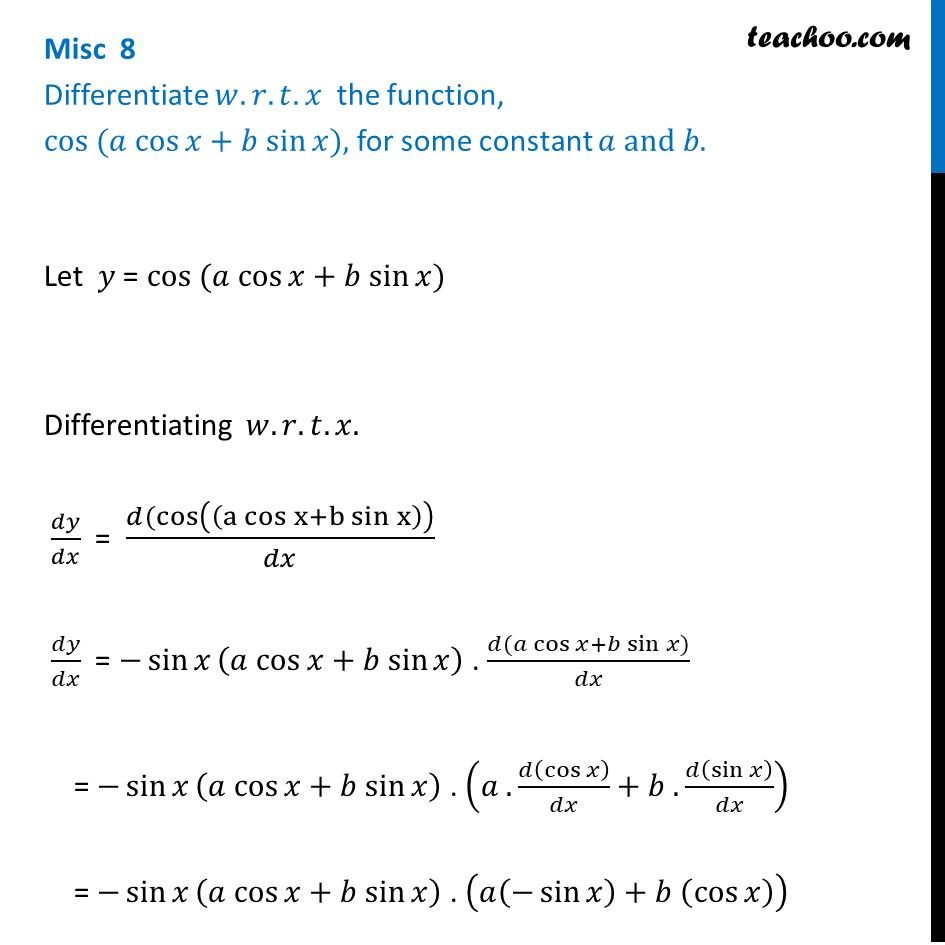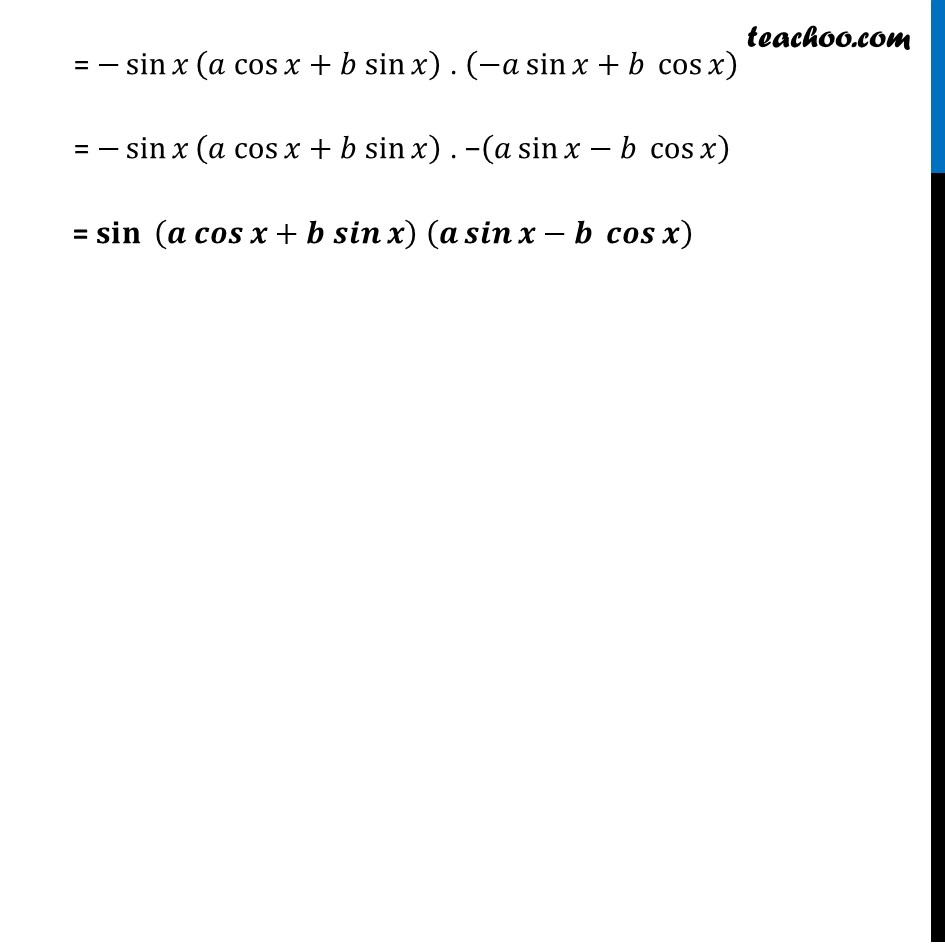Finding derivative of a function by chain rule

Chapter 5 Class 12 Continuity and Differentiability
Concept wiseLearn in your speed, with individual attention - Teachoo Maths 1-on-1 Class

### Transcript

Misc 8 Differentiate 𝑤.𝑟.𝑡. 𝑥 the function, cos ⁡(𝑎 cos⁡𝑥+𝑏 sin⁡𝑥), for some constant 𝑎 and 𝑏. Let 𝑦 = cos ⁡(𝑎 cos⁡𝑥+𝑏 sin⁡𝑥) Differentiating 𝑤.𝑟.𝑡.𝑥. 𝑑𝑦/𝑑𝑥 = (𝑑(cos((a cos⁡x+b sin⁡x )))/𝑑𝑥 𝑑𝑦/𝑑𝑥 = −sin⁡𝑥 (𝑎 cos⁡𝑥+𝑏 sin⁡𝑥) . (𝑑⁡(𝑎 cos⁡𝑥+𝑏 sin⁡𝑥))/𝑑𝑥 = −sin⁡𝑥 (𝑎 cos⁡𝑥+𝑏 sin⁡𝑥) . (𝑎 .𝑑⁡(cos⁡𝑥 )/𝑑𝑥+𝑏 .𝑑⁡(sin⁡𝑥 )/𝑑𝑥) = −sin⁡𝑥 (𝑎 cos⁡𝑥+𝑏 sin⁡𝑥) . (𝑎(−sin⁡𝑥 )+𝑏 (cos⁡𝑥 )) = −sin⁡𝑥 (𝑎 cos⁡𝑥+𝑏 sin⁡𝑥) . (−𝑎 sin⁡𝑥+𝑏 cos⁡𝑥 ) = −sin⁡𝑥 (𝑎 cos⁡𝑥+𝑏 sin⁡𝑥) . −(𝑎 sin⁡𝑥−𝑏 cos⁡𝑥 ) = 𝐬𝐢𝐧⁡〖 (𝒂 𝒄𝒐𝒔⁡𝒙+𝒃 𝒔𝒊𝒏⁡𝒙)" " (𝒂 𝒔𝒊𝒏⁡𝒙−𝒃 𝒄𝒐𝒔⁡𝒙 )〗